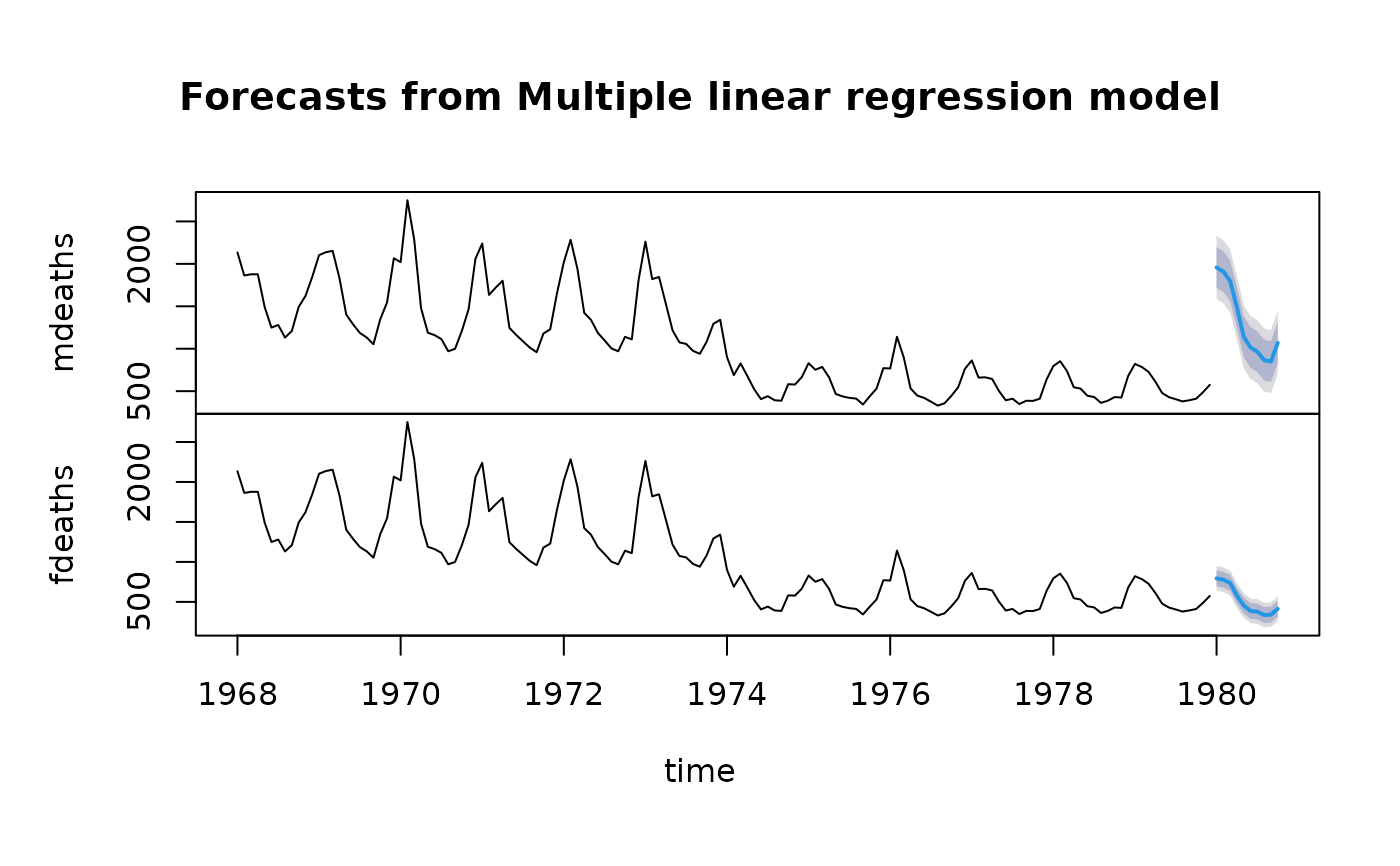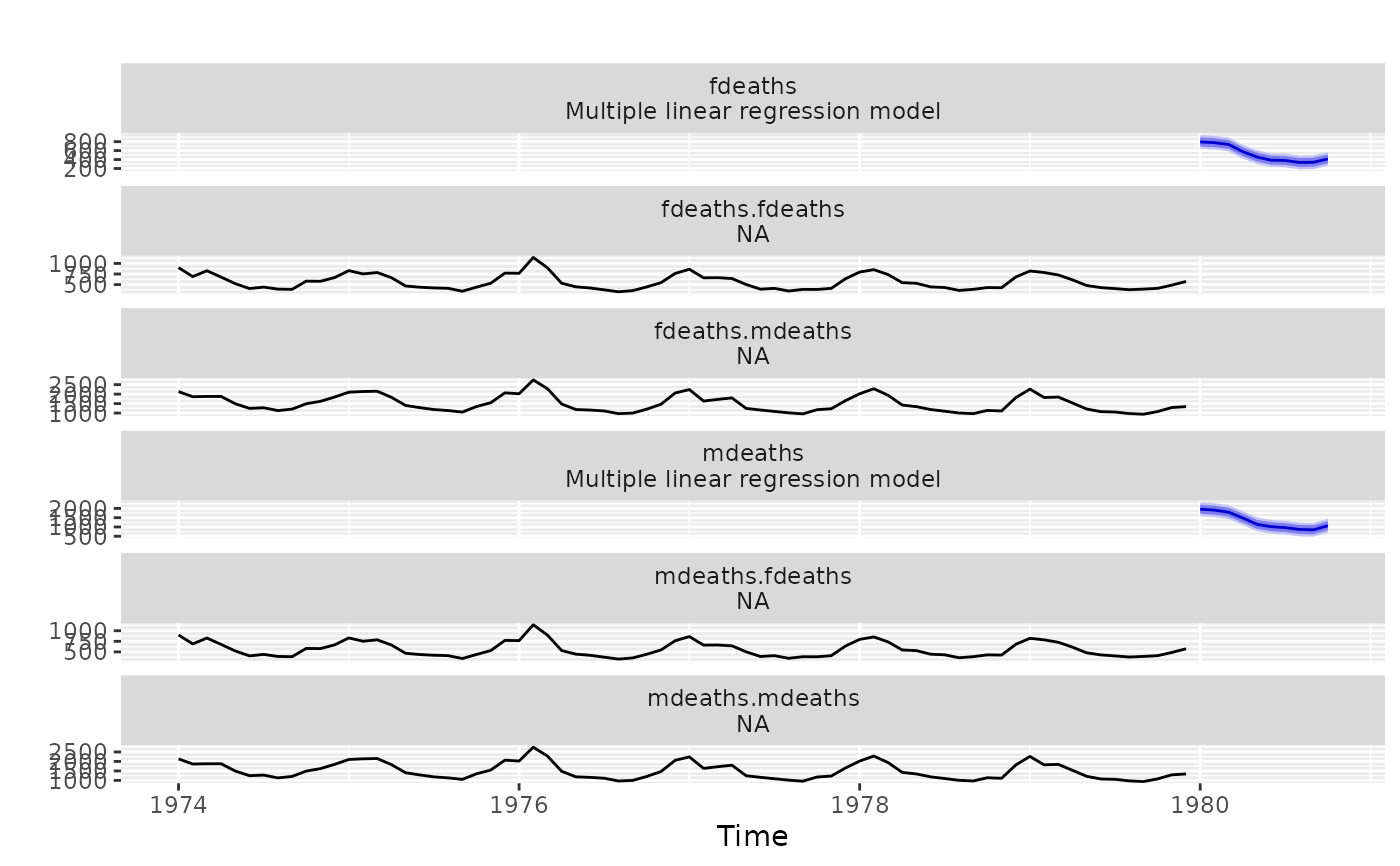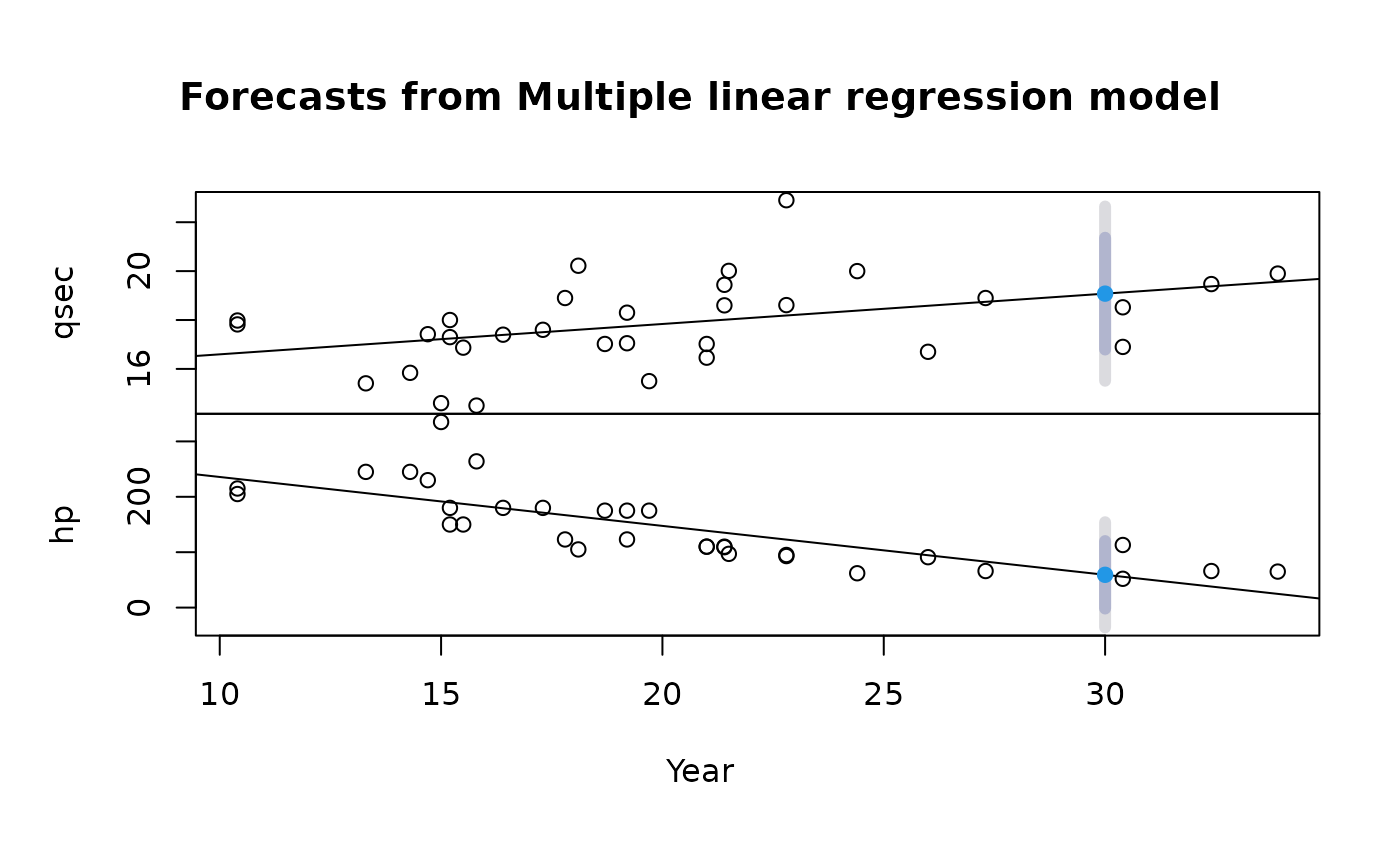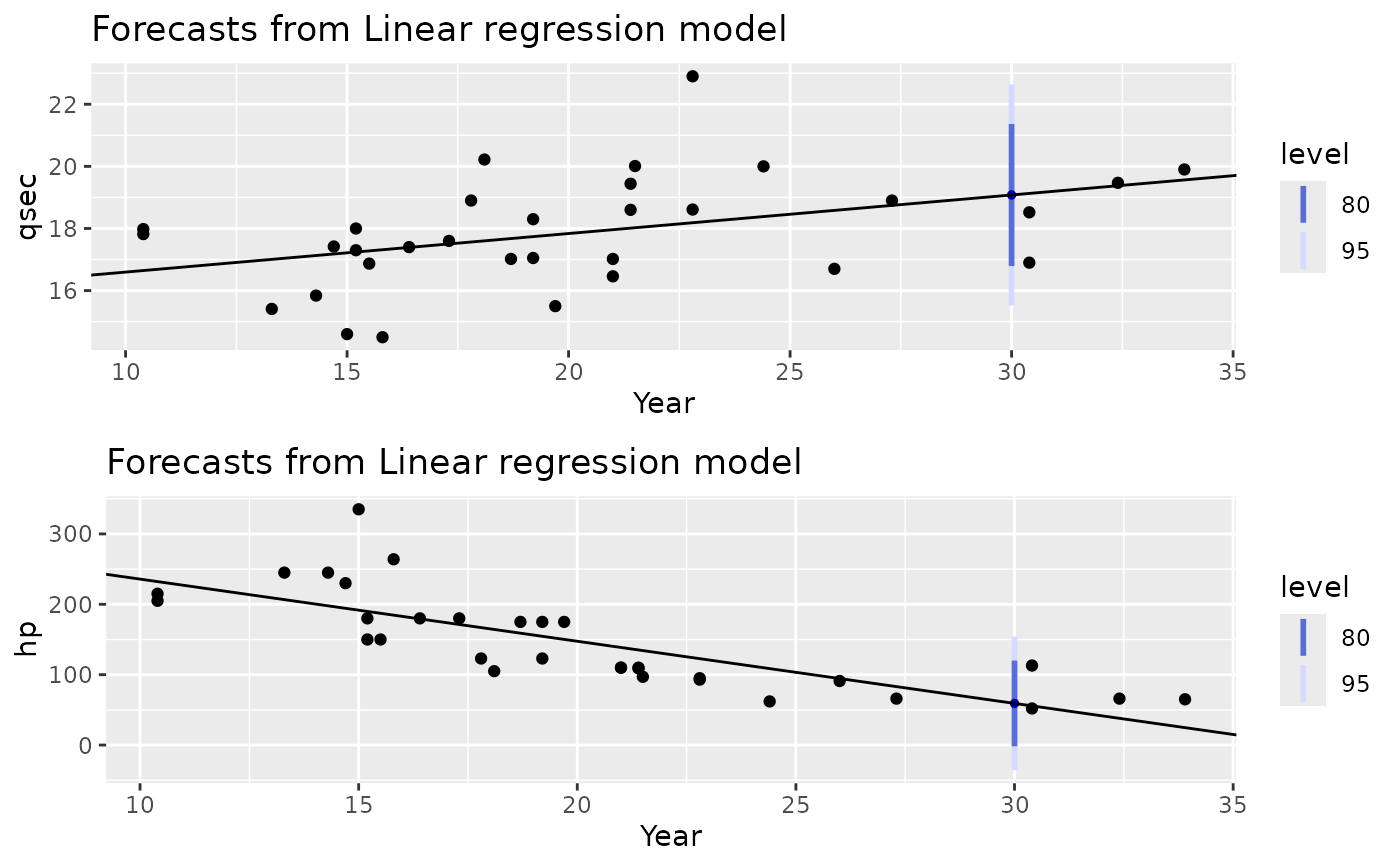Plots historical data with multivariate forecasts and prediction intervals.

# S3 method for mforecast
autoplot(object, PI = TRUE, facets = TRUE, colour = FALSE, ...)

# S3 method for mforecast
autolayer(object, series = NULL, PI = TRUE, ...)

# S3 method for mforecast
plot(x, main = paste("Forecasts from", unique(x\$method)), xlab = "time", ...)

## Arguments

object Multivariate forecast object of class mforecast. Used for ggplot graphics (S3 method consistency). If FALSE, confidence intervals will not be plotted, giving only the forecast line. If TRUE, multiple time series will be faceted. If FALSE, each series will be assigned a colour. If TRUE, the time series will be assigned a colour aesthetic additional arguments to each individual plot. Matches an unidentified forecast layer with a coloured object on the plot. Multivariate forecast object of class mforecast. Main title. Default is the forecast method. For autoplot, specify a vector of titles for each plot. X-axis label. For autoplot, specify a vector of labels for each plot.

## Details

autoplot will produce an equivalent plot as a ggplot object.

Hyndman and Athanasopoulos (2018) Forecasting: principles and practice, 2nd edition, OTexts: Melbourne, Australia. https://otexts.com/fpp2/

plot.forecast, plot.ts

## Author

Mitchell O'Hara-Wild

## Examples

library(ggplot2)

lungDeaths <- cbind(mdeaths, fdeaths)
fit <- tslm(lungDeaths ~ trend + season)
fcast <- forecast(fit, h=10)
plot(fcast)autoplot(fcast)carPower <- as.matrix(mtcars[,c("qsec","hp")])
carmpg <- mtcars[,"mpg"]
fit <- lm(carPower ~ carmpg)
fcast <- forecast(fit, newdata=data.frame(carmpg=30))
plot(fcast, xlab="Year")autoplot(fcast, xlab=rep("Year",2))